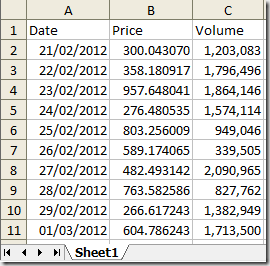### Create excel spreadsheet with C#

```[Test]
public void Test()
{
var file = @"Sample.xlsx";
if (File.Exists(file)) File.Delete(file);
using (var excel = new ExcelPackage(new FileInfo(file)))
{
ws.Cells[1, 1].Value = "Date";
ws.Cells[1, 2].Value = "Price";
ws.Cells[1, 3].Value = "Volume";
var random = new Random();
for (int i = 0; i < 10; i++)
{
ws.Cells[i + 2, 1].Value = DateTime.Today.AddDays(i);
ws.Cells[i + 2, 2].Value = random.NextDouble() * 1e3;
ws.Cells[i + 2, 3].Value = random.Next() / 1e3;
}

ws.Cells[2, 1, 11, 1].Style.Numberformat.Format = "dd/MM/yyyy";
ws.Cells[2, 2, 11, 2].Style.Numberformat.Format = "#,##0.000000";
ws.Cells[2, 3, 11, 3].Style.Numberformat.Format = "#,##0";
ws.Column(1).AutoFit();
ws.Column(2).AutoFit();
ws.Column(3).AutoFit();
excel.Save();
}
}```1.To avoid the coding and handling the errors you can use this Excel Component in C# which not only allow you to create excel file in c#, .net, vb.net but also you can read and convert your excel files to many other formats.

2.I am attracted by the presentation of this article. It is a genuinely a gainful article for us. Keep posting, Thank you.Microsoft Project Basics